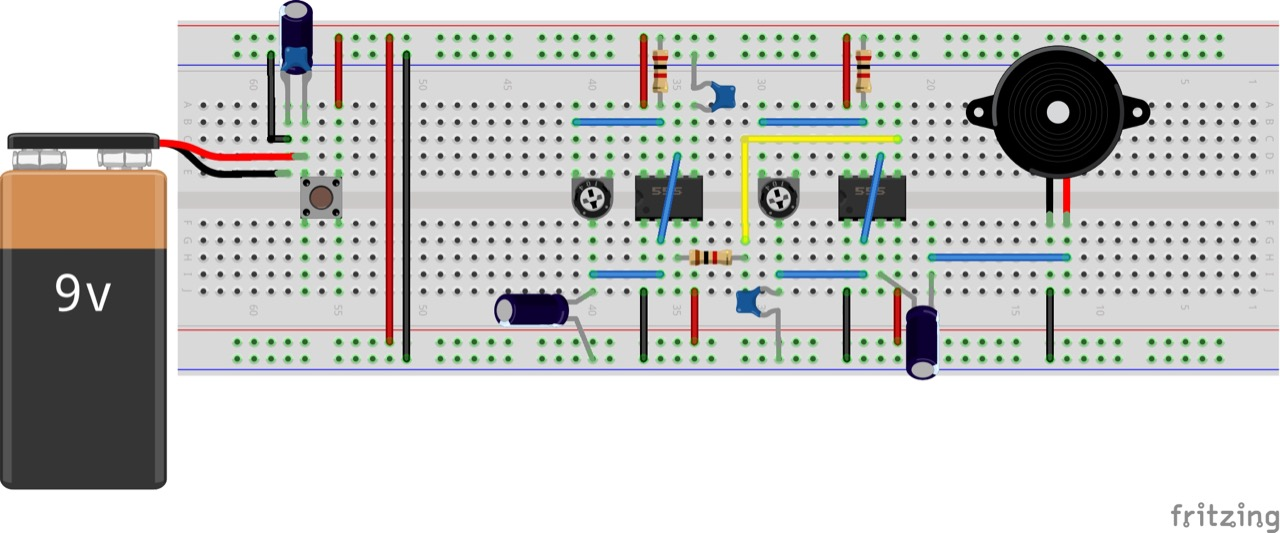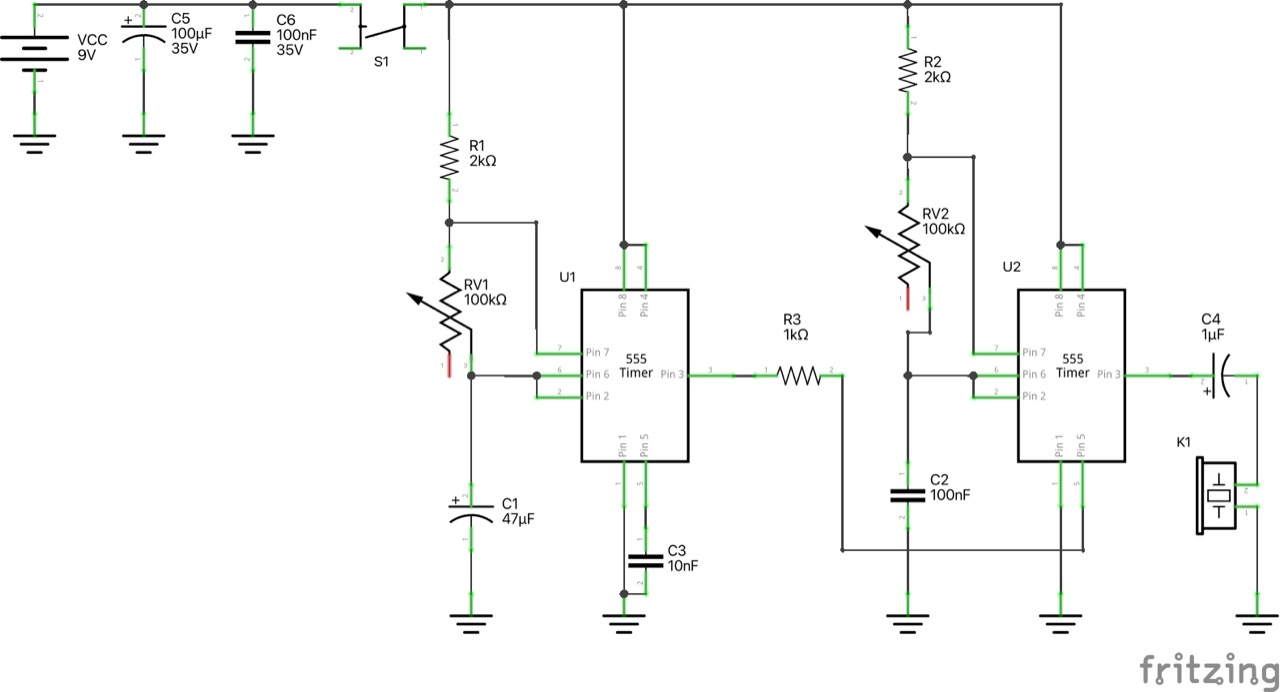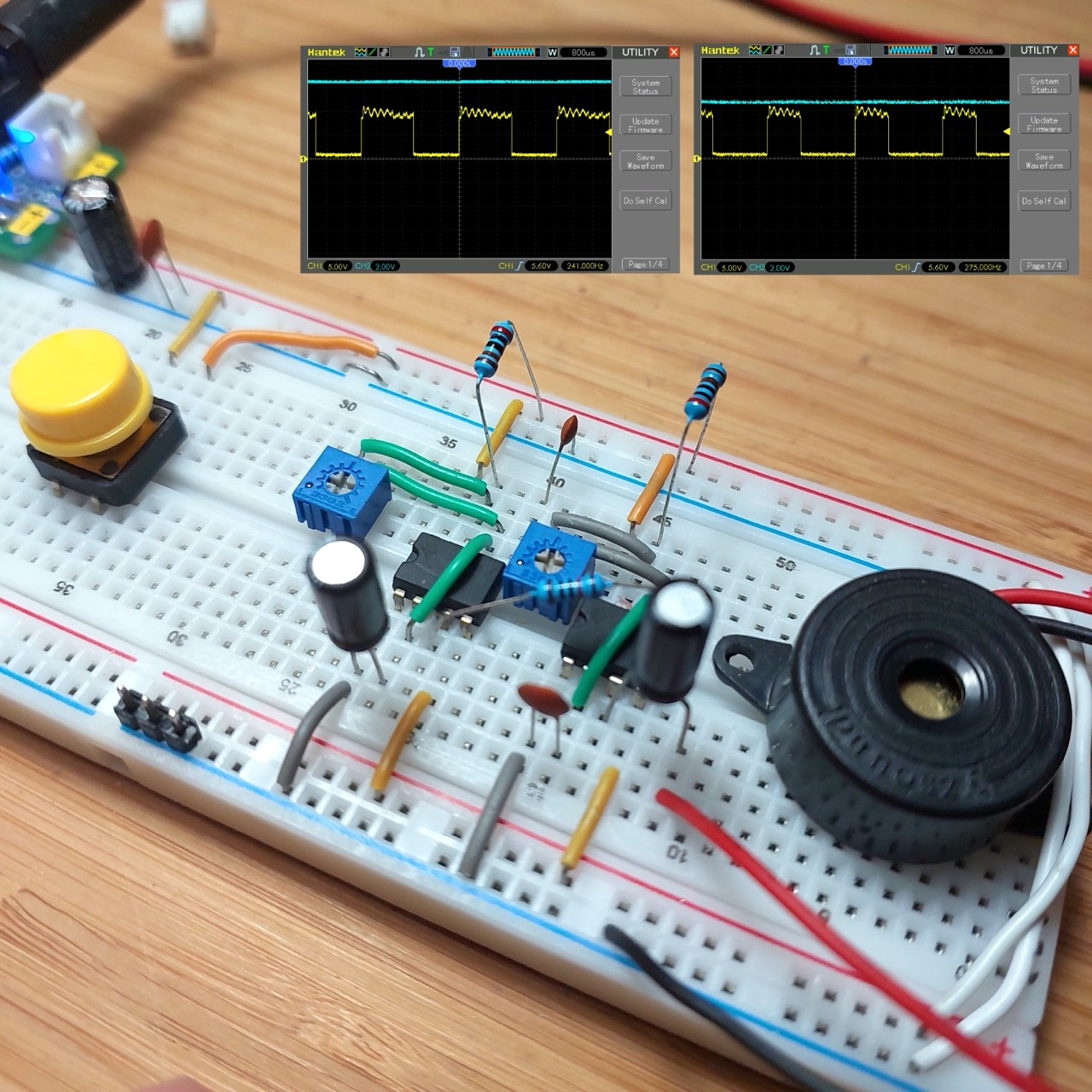# #045 SimpleSiren

Classic timer circuit producing a two-tone oscillation.

It is allegedly a “ding dong” bell.. sounds more like a siren to me!

It operates with two oscillating 555 timers chained together.

The first 555 provides the low frequency oscillation between high and low output states. The frequency is determined by the values of R1, VR1 and C1. With R1=2.2kΩ, VR1 at 22kΩ and C1=47μF this runs at 0.7Hz and 52% duty cycle.

The pin 3 output of the first time switches the control voltage of the second 555 timer. In practice, with R3=1kΩ in place, it switches from 1.57V to 7.06V, as measured with a multimeter.

The oscilation of the second 555 drives the output peizo, and the frequency is modified by the control voltage.

1.57 high 7.06 low

When Vcontrol = 1.57V, the frequency of the oscillation is 566 Hz - see the actual calculation on WolframAlpha

When Vcontrol = 7.06V, the frequency of the oscillation reduces to 248 Hz - see the actual calculation on WolframAlpha.

The derivation of the fomula considering a control voltage is explained in a question on EE.SE. The formula with variables as named in this circuit is:

``````f = 1/( C2.(R2+VR2)ln(1 + Vcontrol/(2(Vcc - Vcontrol))) + C2.VR2.ln(2) )
``````

### Construction